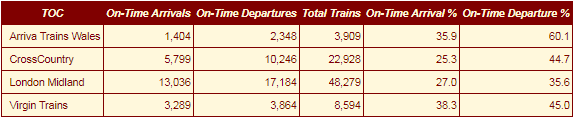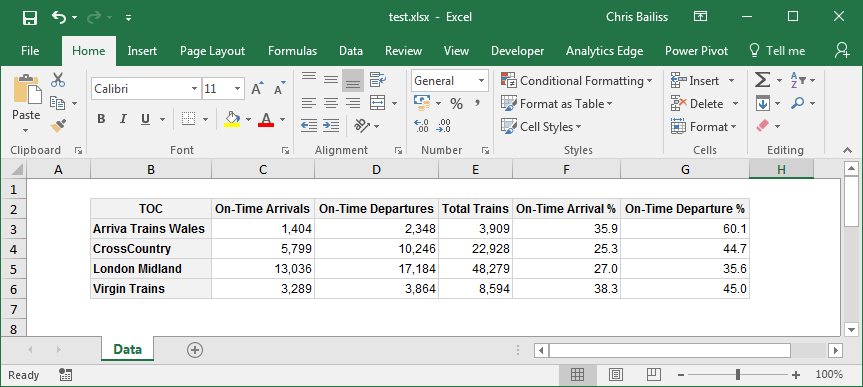The basictabler package enables rich tables to be created and rendered/exported with just a few lines of R.

The basictabler package:

• Provides an easy way of creating basic tables, especially from data frames and matrices.
• Provides flexibility so that the structure/content of the table can be easily built/modified.
• Provides formatting options to simplify rendering/exporting data.
• Provides styling options so the tables can be themed/branded as needed.

The tables are rendered as htmlwidgets or plain text. The HTML/text can be exported for use outside of R.

The tables can also be exported to Excel, including the styling/formatting. The formatting/styling is specified once and can then be used when rendering to both HTML and Excel - i.e. it is not necessary to specify the formatting/styling separately for each output format.

Using the flextabler package it is also possible to output tables to Word and PowerPoint.

basictabler is a companion package to the pivottabler package. pivottabler is focussed on generating pivot tables and can aggregate data. basictabler does not aggregate data but offers more control of table structure.

### Installation

You can install:

• the latest released version from CRAN with
install.packages("basictabler")
• the latest development version from github with
devtools::install_github("cbailiss/basictabler", build_vignettes = TRUE)

### Examples

#### Trivial Example

Creating a tiny HTML table from a data frame and immediately rendering it as a htmlwidget:

library(basictabler)
qhtbl(data.frame(a=1:2, b=3:4))

#### Another Example

Creating a table from a data frame, specifying column names and value formats:

# aggregate the sample data to make a small data frame
library(basictabler)
library(dplyr)
tocsummary <- bhmsummary %>%
group_by(TOC) %>%
summarise(OnTimeArrivals=sum(OnTimeArrivals),
OnTimeDepartures=sum(OnTimeDepartures),
TotalTrains=sum(TrainCount)) %>%
ungroup() %>%
mutate(OnTimeArrivalPercent=OnTimeArrivals/TotalTrains*100,
OnTimeDeparturePercent=OnTimeDepartures/TotalTrains*100) %>%
arrange(TOC)

# To specify formatting, a list is created which contains one element for each column in
# the data frame, i.e. tocsummary contains six columns so the columnFormats list has six elements.
# The values in the first column in the data frame won't be formatted since NULL has been specified.
# The values in the 2nd, 3rd and 4th columns will be formatted using format(value, big.mark=",")
# The values in the 5th and 6th columns will be formatted using sprintf(value, "%.1f")
columnFormats=list(NULL, list(big.mark=","), list(big.mark=","), list(big.mark=","), "%.1f", "%.1f")

# render the table directly as a html widget
"Total Trains", "On-Time Arrival %", "On-Time Departure %"),
columnFormats=columnFormats)In the example above, the qhtbl() functions returns a html widget that is rendered immediately in the R-Studio viewer window. An alternative is to use the qtbl() function which returns a BasicTable object that can be further manipulated. The styling example further below demonstrates this.

#### Changing a Table Example

Tables can also be built row-by-row, column-by-column and cell-by-cell. Once built tables can be modified (adding/removing rows columns and cells, merging cells and changing styling). The following example shows more granular ways of building and changing a table:

# data for the table
saleIds <- c(5334, 5336, 5338)
items <- c("Apple", "Orange", "Banana")
quantities <- c(5, 8, 6)
prices <- c(0.34452354, 0.4732543, 1.3443243)

# construct a table column by column
library(basictabler)
tbl <- BasicTable$new() tbl$cells$setCell(1, 1, cellType="root", rawValue="Sale ID") tbl$cells$setCell(1, 2, cellType="columnHeader", rawValue="Item") tbl$cells$setCell(1, 3, cellType="columnHeader", rawValue="Quantity") tbl$cells$setCell(1, 4, cellType="columnHeader", rawValue="Price") tbl$cells$setColumn(1, cellTypes="rowHeader", rawValues=saleIds) tbl$cells$setColumn(2, cellTypes="cell", rawValues=items) tbl$cells$setColumn(3, cellTypes="cell", rawValues=quantities) tbl$cells$setColumn(4, cellTypes="cell", rawValues=prices, formats=list("%.2f")) # example of changing the table - appending a row formats <- list(NULL, NULL, NULL, "%.2f") cellTypes=c("rowHeader", "cell", "cell", "cell") tbl$cells$setRow(5, cellTypes=cellTypes, formats=formats, rawValues=list(5343, "Pear", 2, 1.0213424)) # example of changing the table - inserting a row tbl$cells$insertRow(1) tbl$cells$setRow(1, cellTypes="columnHeader", rawValues=list("Sale ID", "Sale Details", "", "")) # example of changing the table - merging some cells tbl$mergeCells(rFrom=1, cFrom=1, rSpan=2, cSpan=1)
tbl$mergeCells(rFrom=1, cFrom=2, rSpan=1, cSpan=3) # render the final table tbl$renderTable()#### Styling Example

Styling can be specified when creating tables:

# aggregate the sample data to make a small data frame
library(basictabler)
library(dplyr)
tocsummary <- bhmsummary %>%
group_by(TOC) %>%
summarise(OnTimeArrivals=sum(OnTimeArrivals),
OnTimeDepartures=sum(OnTimeDepartures),
TotalTrains=sum(TrainCount)) %>%
ungroup() %>%
mutate(OnTimeArrivalPercent=OnTimeArrivals/TotalTrains*100,
OnTimeDeparturePercent=OnTimeDepartures/TotalTrains*100) %>%
arrange(TOC)

# column formats
columnFormats=list(NULL, list(big.mark=","), list(big.mark=","), list(big.mark=","), "%.1f", "%.1f")

# create the table
"Total Trains", "On-Time Arrival %", "On-Time Departure %"),
columnFormats=columnFormats,
tableStyle=list("border-color"="maroon"),
"font-style"="italic", "border-color"="maroon"),
cellStyle=list("color"="maroon", "background-color"="cornsilk",
"border-color"="maroon"))

# set column alignment of first column
# the arguments are (rFrom, cFrom, rTo, cTo, declarations)
tblsetStyling(2, 1, 5, 1, declarations=list("text-align"="left")) # render table tblrenderTable()#### Excel Output

The same styling/formatting used for the HTML output is also used when outputting to Excel - greatly reducing the amount of script that needs to be written to create Excel output. The only additional formatting that typically needs applying is the Excel cell format strings.

# aggregate the sample data to make a small data frame
library(basictabler)
library(dplyr)
tocsummary <- bhmsummary %>%
group_by(TOC) %>%
summarise(OnTimeArrivals=sum(OnTimeArrivals),
OnTimeDepartures=sum(OnTimeDepartures),
TotalTrains=sum(TrainCount)) %>%
ungroup() %>%
mutate(OnTimeArrivalPercent=OnTimeArrivals/TotalTrains*100,
OnTimeDeparturePercent=OnTimeDepartures/TotalTrains*100) %>%
arrange(TOC)

columnFormats=list(NULL, list(big.mark=","), list(big.mark=","), list(big.mark=","), "%.1f", "%.1f")

# create the table
"Total Trains", "On-Time Arrival %", "On-Time Departure %"),
columnFormats=columnFormats)

# set the styling on the count cells
# the arguments are (rFrom, cFrom, rTo, cTo, declarations)
tbl$setStyling(2, 2, 5, 4, declarations=list("xl-value-format"="#,##0")) # set the styling on the average delay cells tbl$setStyling(2, 5, 5, 6, declarations=list("xl-value-format"="##0.0"))

# render the table to an Excel workbook
library(openxlsx)
tbl\$writeToExcelWorksheet(wb=wb, wsName="Data",
topRowNumber=2, leftMostColumnNumber=2, applyStyles=TRUE)
saveWorkbook(wb, file="C:\\test.xlsx", overwrite = TRUE)In the screenshot above, Gridlines have been made invisible to make the styling easier to see (by clearing the checkbox on the ‘View’ ribbon). Columns were also auto-sized - though the widths of columns could also be manually specified from R. See the Excel Export vignette for more details.

It is possible to create tables from data frames, matrices, row-by-row, column-by-column and/or cell-by-cell.

Tables can be further manipulated once created, including adding/removing cells/rows/columns and merging cells.

Styling and formatting can be specified for individual cells and ranges of cells.

# to see a list of available package vignettes:
vignette(topic="v01-introduction", package="basictabler")